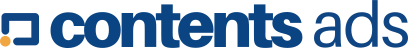# How much does one gallon of water weigh?

History of a gallon

A gallon is a measure of volume, equating to either 3.79L or 4.55L depending on its usage.

The imperial (UK) gallon is equal to 4.546 L. It was made obsolete through The Units of Measurement Regulations 1995 (EU Directive 80/181/EEC).

This effectively outlawed imperial measurements, replacing them with metric measurements.

A US liquid gallon equates to 3.785 L and is accepted as the definition of a gallon in the US and other countries such as Colombia, Ecuador, Haiti and Peru.

The gallon can be divided into eight pints or four quarts. It can also be further divided into gills. With the imperial measurements being non decimal it was rather difficult to convert between different units (eg. from pints to gallons). In the twentieth century the metric system was introduced with the aim of producing an internationally acceptable unit of measurement.

The significance of the imperial/metric system is that when posed with the question of ‘how much does a gallon of water weigh‘ it needs to be taken into consideration which type of gallon is being referred to; the US liquid gallon or the UK gallon.

Converting gallons using the US liquid gallon

Calculating the weight of one gallon of water first involves using the conversion factor of 8.33. This value means that one gallon of water will weigh 8.33 pounds. 10 gallons of water will weigh 83.30 pounds.

If you were to convert one US liquid gallon into metric units you would use the factor of 3.785. One US liquid gallon would equal to 3.785 L.

Converting gallons using the imperial (UK) gallon

The UK gallon has a factor of 10.022. Therefore one gallon would equal 10.022 pounds. Its metric counterpart has a factor of 4.546 and therefore one gallon would equal to 4.546 kg.

You could also convert the volume to metric units first (i.e. gallon to litre) and then convert to weight. Since 1 L of water = 1kg the factor of 4.546 would still hold true.

Weight of other liquids

One gallon of water will not weigh the same as one gallon of gas (gasoline). This is because gasoline is 0.72 times less dense compared to water.

Gasoline weighs 0.72 kg per L.

Using the value from above. 4.546 L (i.e. one imperial gallon) of gasoline would equal 3.27 kg The density of liquids also varies with a change in temperature.

Inline FeedbacksContacts:

### When Biomedical Science Meets Strategic Partnering: Eyram Adjogatse on the Future of Pharmacological Discoveries

13 November, 2023

### International Association of Honored Developers’ Participants Number Has Grown By 50% in 2023!

9 November, 2023
The growth of the International Association of Honored Developers.

### The future of working remotely in 2023 and expectations

21 February, 2023
Many companies during lockdown had to incorporate working remotely in their lifestyle, and even though smart working isn’t a necessity anymore, studies have indicated that the demand of this way…